# Microelectronics

## 09 VHDL, FPGA and Delay

### Prof. Dr. Jörg Vollrath

08 System, Synthesis, VHDL

## Video of lecture 10 (2.6.2021)

 Video is not visible, most likely your browser does not support HTML5 video Länge: 0:55:04 0:0:0 Timing Closure and Delay 0:2:30 tDelay 0:7:30 Power consumption 0:13:30 Delay in and out 0:16:0 Delay equation with load L 0:17:43 Explanation of schematic 0:19:20 Delay equation with load L and driver W 0:21:20 Plotted graph discussion 0:26:37 Delay with second inverter, 2 stages 0:28:10 Explanation of delay 0:29:16 tDelay with 2 stages 0:32:22 Final equation 0:34:43 Graph discussion 0:39:25 Unit transistor scaling with number of inputs 0:41:53 Question 0:44:57 Equation start 0:47:23 Graph discussion 0:49:59 Simplistic model no output capacitance 0:53:5 Calculation of delay in JavaScript

# Overview

Review:
• Unit transistors, cell layout
• System synthesis
• VHDL entity and architecture

Today:

• VHDL, VHDL books
• ASIC, FPGA, microprocessor
• Delay and Timing Closure: Inverter sizing, pipeline

# Systems

## FPGA

Field programmable gate array (FPGA)

• Programmable
• Switch matrix
• Logic
• Registers
• Microprocessor
• Design entry
• VHDL, Verilog
• Schematic
• State diagram
• Matlab, Labview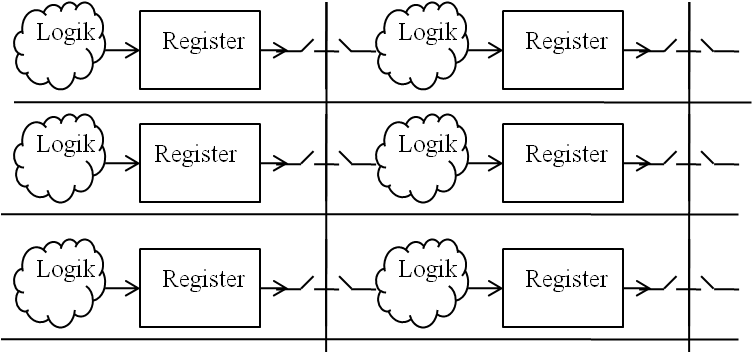Switch matrix, logic and registers, programmable

# Xilinx FPGA configurable lookup table

## Logic, register (folder: netgen/synthesis

  curr_zero_not000125 : LUT4 generic map( INIT => X"135F" ) port map ( I0 => curr_ir(3), I1 => curr_ir(2), I2 => curr_acc(3), I3 => curr_acc(2), O => curr_zero_not000125_120 ); Logic: AND INIT => X“8000“ OR INIT => X“FFFE“ RAM: I0..I3: address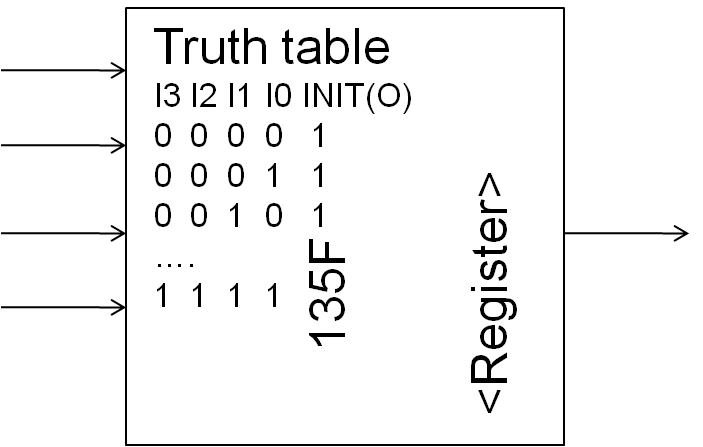# Books: FPGA Programming and VHDL

## Rapid Prototyping of Digital Systems:

Quartus® II Edition
Hamblen
71.- Euro

## FPGA Prototyping by VHDL Examples:

Xilinx Spartan-3 Version
Chu
68.99.-EuroNo cost VHDL compiler and simulator:
FPGA vendors: Intel(Altera), Xilinx

# Microelectronics Design Optimization

• Technology:
• FPGA, Gate Array, Standard cells, Full custom
• Design
• SystemC, VHDL/Verilog (RTL), Schematic
• Block technology:
• CPU, State machine
• Synchronous logic, asynchronous logic
• Memory: SRAM, RAM, NAND Flash
• CMOS logic style
• AOI, dynamic, transfer gate (TG), dynamic CMOS, domino
• Layout style
• Regular, full custom, transistor sizing

# Gate Array, Semi-Custom, and Full-Custom ICs

Gate Array (biggest): Everything is prefabricated except metal connection
Standard cells: Logic cells are placed and routed
Full custom (smallest): Each gate is individually drawn and routed.

## Area ratio: 3:2:1# Timing Closure and Propagation Delay

Measuring propagation delay requires a real driver to measure the influence of input capacitance and a real capacitance load to be charged. In the center is the DUT (device under test).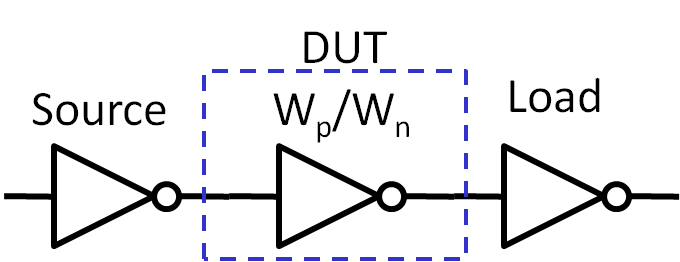• Propagation Delay:    $t_{delay} = 0.7 R C_{tot}$
R: on resistance of PFET/NFET Ron;    C: load capacitance
• Changing Wp/Wn
Input capacitance changes, Ron changes
Smaller Ron and bigger input capacitance
How to optimize timing performance?
Timing closure
No premature optimization!

# Propagation Delay• Propagation Delay
• Source inverter, DUT and load inverter minimum size: R, C
• tdelay = tdelayin + tdelayout = 0.7 R CDUTin + 0.7 RDUTon C
• R: on resistance of PFET/NFET
• Changing all Wp/Wn
• Increasing width: Rnew= R/2, Cnew= 2 C
• tdelay = tdelayin + tdelayout = 0.7 R/2 2 CDUTin + 0.7 RDUTon/2 2 C
• No change in speed, higher power consumption, more area

# Topology and Propagation Delay

 Inverter chain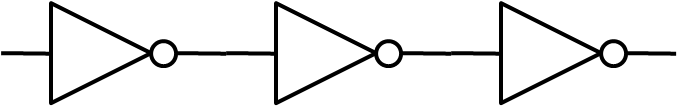Load: Driving a line or multiple logic gates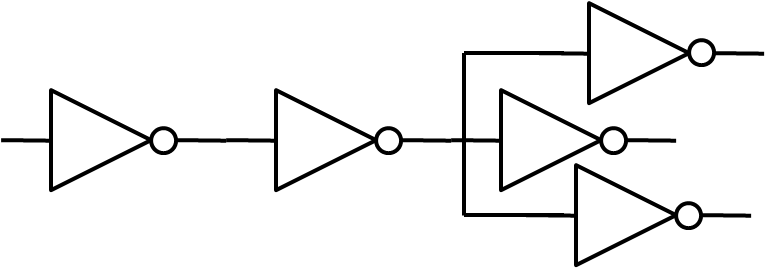Transistors in series or parallel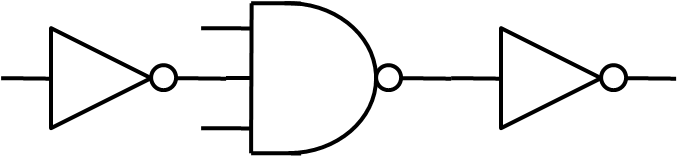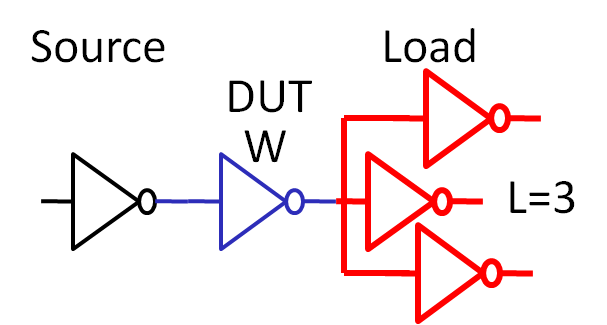Load (L): Driving a line or multiple logic gates
tdelay = tdelayin + tdelayout = 0.7 R · (W · C) + 0.7 (R/W) (L · C)
tdelay = 0.7 R · C ( W + L/W) = tdelay0 ( W + L/W)

Delay with large loads can be reduced with width W.
Using unit transistors W should be an integer number.
tdelay(L=1) = tdelay ( W + 1/W)
tdelay(L=5) = tdelay ( W + 5/W)
tdelay(L=10) = tdelay ( W + 10/W)

### Power

PAVG= VDD · IAVG
Current is needed to charge and discharge capacitances.
$C = \frac{Q}{V} = \frac{I \cdot t}{V}$
$I = \frac{C \cdot V}{t} = C \cdot V_{DD} \cdot f_{CLK}$
PAVG= VDD2 · C · fCLK

This is the active power consumption.
There are also cross currents from VDD to gnd during switching and transistor leakage currents.

Changing W to larger values increases power consumption.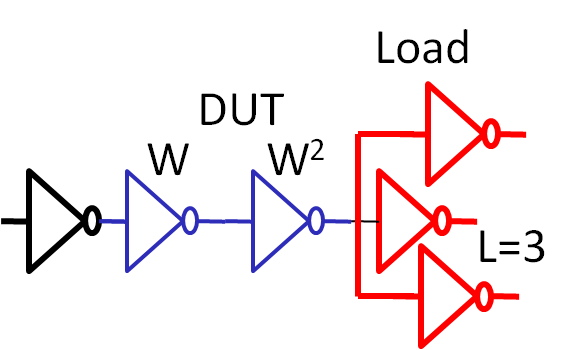Load: Driving a line or multiple logic gates with 2 stages
tdelay = tdelayin + tmid + tdelayout = 0.7 R W CDUTin + 0.7 RDUTon/W W * W C + 0.7 RDUTon/W/W L C = tdelay ( W + W + L/W/W)

For higher loads use more than one stage.

# Propagation Delay and Logic function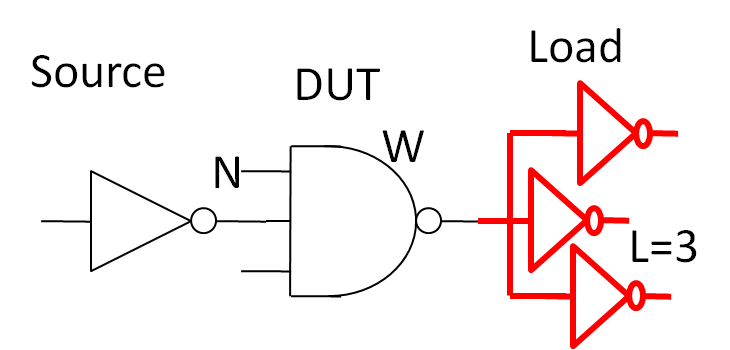N inputs: N transistors in series in logic block
tdelay = tdelayin + tdelayout = 0.7 R W CDUTin + 0.7 N RDUTon / W * C * L = tdelay ( W + N / W * L)

Change only width W of series transistors
tdelay1 = tdelayin + tdelayout = 0.7 R (0.5 + 0.5 W ) CDUTin + 0.7 N RDUTon/ W C * L = tdelay ( 0.5 + 0.5 * W + N / W *L )

# Propagation Delay and Pipelines

What is the maximum clock frequency?
tpd = 5 ns, tsetup = 1 ns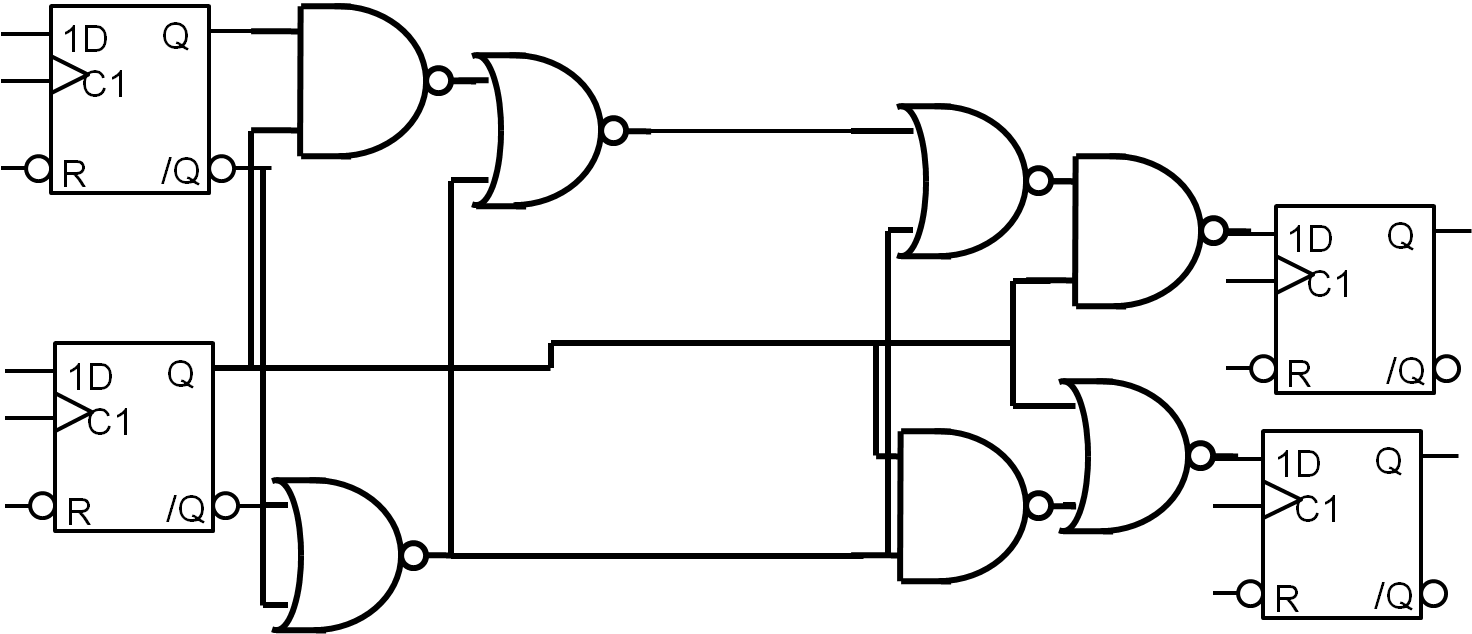# Retiming of synchronous logic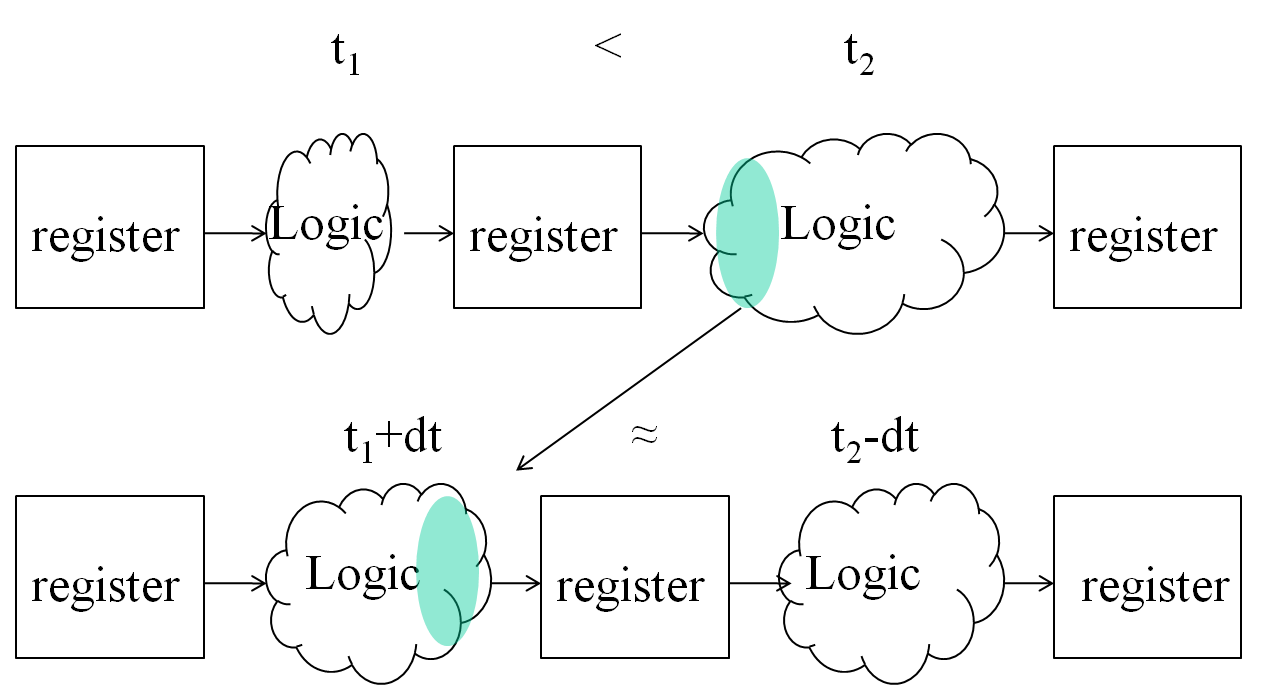# FPGA Timing

• Provide VHDL code to realize pipeline
Registers only
• Retiming done by compiler
• Input CLK should use internal PLL to avoid setup and hold issues

# Summary

• Design strategy
• FPGA, gate array, full custom design
• ## High level language (SystemC, VHDL) and synthesis of layout

• Timing closure
• Transistor resizing